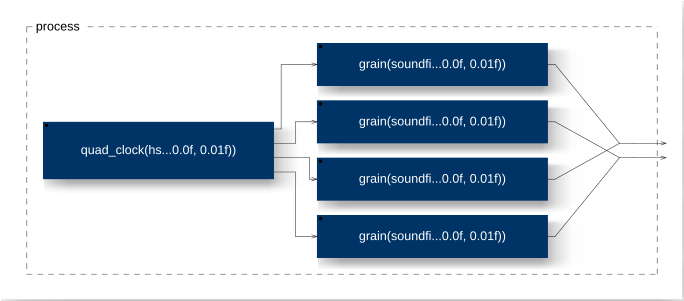# Granular: Faust Example

The grain_player.dsp example in the repository uses four parallel grain processes, as shown in [Fig.1].Fig.1

Four parallel grain players

The code below does not handle all problem cases. Depending on the sound material, changing the grain position may result in audible clicks. For high densities, grains are retriggered before their ampltude dacays to 0 - also resulting in clicks.

// grain_player.dsp
//
// Play a wave file in grains.
//
// - four grains
// - glitches when changing grain position
//
// Henrik von Coler
// 2020-05-28

import("stdfaust.lib");

// read a set of wav files
s = soundfile("label[url:{'../WAV/chips.wav';   '../WAV/my_model.wav'; '../WAV/sine.wav'}]", 1);

// a slider for selecting a sound file:
file_idx = hslider("file_idx",0,0,2,1);

// a slider for controlling the playback speed of the grains:
speed = hslider("speed",1,-10,10,0.01);

// start point for grain playback
start = hslider("start",0,0,1,0.01);

// a slider for the grain length:
length = hslider("length",1000,1000,40000,1): si.smoo;

// control the sample density (or the clock speed)
density = hslider("density", 0.1,0.01,20,0.01);

// the ramp is used for scrolling through the indices
ramp(f, t) = delta : (+ : select2(t,_,delta<0) : max(0)) ~ _ : raz
with {

// keep below 1:
raz(x) = select2 (x > 1, x, 0);
delta = sh(f,t)/ma.SR;

// sample and hold
sh(x,t) = ba.sAndH(t,x);
};

// 4 impulse trains with 1/4 period phase shifts
quad_clock(d) = os.lf_imptrain(d) <:  _ , ( _ : @(0.25*(1/d) * ma.SR)) , ( _ : @(0.5*(1/d) * ma.SR)), ( _ : @(0.75*(1/d) * ma.SR)) ;

// function for a single grain
grain(s, part, start, l,tt) = (part, pos) : outs(s) : _* win_gain
with {

// ramp from 0 to 1
r = ramp(speed,tt);

// the playback position derived from the ramp
pos = r*l + (start*length(s));

// a simple sine window
win_gain = sin(r*3.14159);

// get recent file's properties
length(s) = part,0 : s : _,si.block(outputs(s)-1);
srate(s)  = part,0 : s : !,_,si.block(outputs(s)-2);
// play sample
outs(s) = s : si.block(2), si.bus(outputs(s)-2);

};

// four parallel grain players triggered by the quad-clock
process =  quad_clock(density) : par(i,4,grain(s, file_idx, start, length)) :> _,_;// :> _ <: _,_;